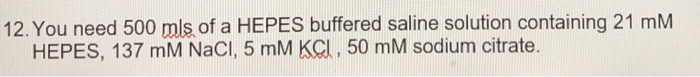# 12. You need 500 mls of a HEPES buffered saline solution containing 21 mM HEPES, 137 mM NaCI, 5 mM KCI, 50 mM sodiu...12. You need 500 mls of a HEPES buffered saline solution containing 21 mM HEPES, 137 mM NaCI, 5 mM KCI, 50 mM sodium citrate.

The necessary moles of each compound are calculated to prepare the solution:

n HEPES = M * V = 0.021 M * 0.5 L = 0.0105 mol

n NaCl = 0.137 M * 0.5 L = 0.0685 mol

n KCl = 0.005 M * 0.5 L = 0.0025 mol

n Citrate = 0.05 M * 0.5 L = 0.025 mol

The required grams of each compound are calculated, according to its molar weight:

g HEPES = n * MM = 0.0105 mol * 238.3 g / mol = 2.50 g

g NaCl = 0.0685 mol * 58.44 g / mol = 4.00 g

g KCl = 0.0025 mol * 74.55 g / mol = 0.19 g

g Citrate = 0.025 mol * 258.1 g / mol = 6.45 g

If you liked the answer, please rate it in a positive way, you would help me a lot, thank you.

##### Add Answer of: 12. You need 500 mls of a HEPES buffered saline solution containing 21 mM HEPES, 137 mM NaCI, 5 mM KCI, 50 mM sodiu...
More Homework Help Questions Additional questions in this topic.